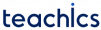# Electromagnetic Flow Meter

Electromagnetic flow meters are suitable for measuring the flow of electrically conducting liquid.

Schematic diagram of an electromagnetic flowmeter is shown in the figure. It consists of a pair of electrodes fixed in the opposite sides of a pipe carrying the fluid whose flow is to be measured. A pipe with non-conducting and non-magnetic property is used. The pipe is surrounded by some material to produce a magnetic field.

An electromagnetic flowmeter works on the Faraday’s Law of Electromagnetic induction. A voltage is produced inside the pipe which is proportional to the velocity of the medium and it is captured by electrodes. The voltage is given byWhere B=Magnetic flux * Density, l=Length of the conductor and v= Velocity of the conductor.

That is if the magnetic field is kept constant then voltage produced across the pipe will be proportional to the velocity of the fluid.

Subscribe
Notify of